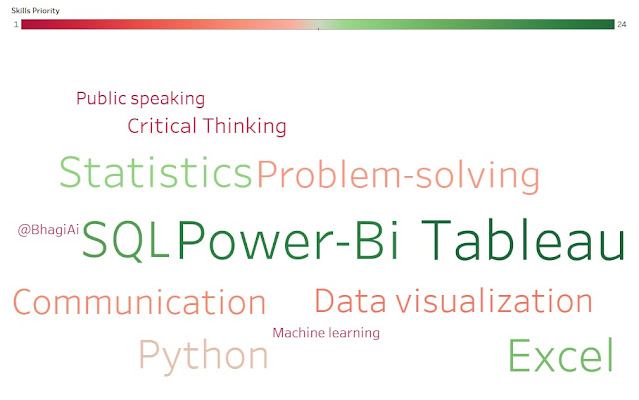## Posts

Showing posts from December, 2022

### SQL Inner Query Alias And Join

use sample_first_db; select * from sample_first_db..customers; select * from sample_first_db..[orders]; -- april may month customers order count --step 1 select *, month([order date]) mnth from orders where month([order date]) in (4,5) --step2 select [customer id], mnth, count(distinct [order id]) cnt from (select *, month([order date]) mnth from orders where month([order date]) in (4,5)) a group by [customer id],mnth --step3 select [customer id],MONTH([order date]) as month, COUNT(DISTINCT [order id]) count from orders  where MONTH([order date]) in (4,5) group by [customer id],MONTH([order date]) select [customer id], case when months = 4 then 'April'  When months = 5 then 'May' end as month_name, counts from (select [customer id], MONTH([order date]) as months,  COUNT(DISTINCT [order id]) counts  from orders  where MONTH([order date]) in (4,5)  group by [customer id],MONTH([order date]))inner_table; -- inner table alias name use. use

### SQL Joins - inner join, left join, right join, full outer join, cross join

drop table if exists table1; create table table1(id VARCHAR(30)); insert into table1 (id) values (1), (2), (3), (4), (5), (null) --insert into table1 values ('hello') --error in joins if in on condition we use this table with other interger value table -- not error if we use only table 2 interger value with integer. table.id = 2;  --like tihs select * from table2 left join table1 on table2.id = 2; select * from table1; drop table if exists table2; create table table2 (id integer); insert into table2 (id) values (2), (2), (6), (4); --insert into table2 (id) values ("hello"); select * from table2; select * from table1; select * from table2; --inner join select * from table1 inner join table2 on table1.id = table2.id; select * from table1,table2 where table1.id = table2.id; select * from table1 cross join table2 where table1.id = table2.id; -- error if we use "on" in crossjoin. use where clause with crossjoin. --iner join on = cross join where = same result se

### The Truth - 3 skills actually required to get Data Analyst job and internship in 2023?There are many misconceptions related to the data analyst job profiles. That you need to learn hardcore programming, maths, and machine learning algorithm to get a data analyst job in 2023. Some people also mix these 3 filed quite frequently. Data Analysis, Data Science, and Data Engineering. Skills to become data analysts in 2023 Excel SQL Tableau/Power-Bi. That's it. And if you have the below skills that would be an advantage. Python Statistics